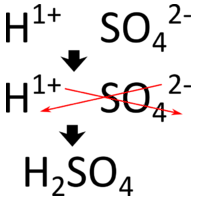7.12: Naming Acids

A spot test for gold has been in use for decades. The sample is first treated with nitric acid. Other metals may react or dissolve in this acid, but gold will not. Then the sample is added to a mixture of nitric acid and hydrochloric acid. Gold will only dissolve in this mixture. The term "acid test" arose from the California gold rush in the late 1840's when this combination was used to test for the presence of real gold. It has since come to mean, "tested and approved" in a number of fields.

Acids

An acid can be defined in several ways. The most straightforward definition is that an acid is a molecular compound that contains one or more hydrogen atoms and produces hydrogen ions $$\left( \ce{H^+} \right)$$ when dissolved in water.Figure $$\PageIndex{1}$$: (A) Vinegar comes in a variety of types, but all contain acetic acid. (B) Citrus fruits like grapefruit contain citric and ascorbic acids.

This is a different type of compound than the others we have seen so far. Acids are molecular, which means that in their pure state they are individual molecules and do not adopt the extended three-dimensional structures of ionic compounds like $$\ce{NaCl}$$. However, when these molecules are dissolved in water, the chemical bond between the hydrogen atom and the rest of the molecule breaks, leaving a positively-charged hydrogen ion and an anion. This can be symbolized in a chemical equation:

$\ce{HCl} \rightarrow \ce{H^+} + \ce{Cl^-}$

Since acids produce $$\ce{H^+}$$, cations upon dissolving in water, the $$\ce{H}$$ of an acid is written first in the formula of an inorganic acid. The remainder of the acid (other than the $$\ce{H}$$) is the anion after the acid dissolves. Organic acids are also an important class of compounds, but will not be discussed here. A binary acid is an acid that consists of hydrogen and one other element. The most common binary acids contain a halogen. An oxoacid is an acid that consists of hydrogen, oxygen, and a third element. The third element is usually a nonmetal.

Naming Acids

Since all acids contain hydrogen, the name of an acid is based on the anion that goes with it. These anions can either be monatomic or polyatomic. The name of all monatomic ions ends in -ide. The majority of polyatomic ions end in either -ate or -ite, though there are a few exceptions such as the cyanide ion $$\left( \ce{CN^-} \right)$$. It is the suffix of the anion that determines how the acid is named as displayed in the rules and table below.

Anion Suffix Example Name of Acid Example
Table $$\PageIndex{1}$$: Naming System for Acids
-ide chloride $$\left( \ce{Cl^-} \right)$$ hydro_____ic acid hydrochloric acid $$\left( \ce{HCl} \right)$$
-ate sulfate $$\left( \ce{SO_4^{2-}} \right)$$ ______ic acid sulfuric acid $$\left( \ce{H_2SO_4} \right)$$
-ite nitrite $$\left( \ce{NO_2^-} \right)$$ ____ous acid nitrous acid $$\left( \ce{HNO_2} \right)$$

The three different suffixes that are possible for the anions lead to the three rules below.

1. When the anion ends in -ide, the acid name begins with the prefix hydro-. The root of the anion name goes in the blank (chlor for chloride), followed by the suffix -ic. $$\ce{HCl}$$ is hydrochloric acid because $$\ce{Cl^-}$$ is the chloride ion. $$\ce{HCN}$$ is hydrocyanic acid because $$\ce{CN^-}$$ is the cyanide ion.
2. When the anion ends in -ate, the name of the acid is the root of the anion followed by the suffix -ic. There is no prefix. $$\ce{H_2SO_4}$$ is sulfuric acid (not sulfic) because $$\ce{SO_4^{2-}}$$ is the sulfate ion.
3. When the anion ends in -ite, the name of the acid is the root of the anion followed by the suffix -ous. Again, there is no prefix. $$\ce{HNO_2}$$ is nitrous acid because $$\ce{NO_2^-}$$ is the nitrite ion.

Note how the root for a sulfur-containing oxoacid is sulfur- instead of just sulf-. The same is true for a phosphorus-containing oxoacid. The root is phosphor- instead of simply phosph-.

Many foods and beverages contain citric acid. Vinegar is a dilute solution of acetic acid. Car batteries contain sulfuric acid that helps in the release of electrons to create electricity.

Writing Formulas for Acids

Like other compounds that we have studied, acids are electrically neutral. Therefore, the charge of the anion part of the formula must be exactly balanced out by the $$\ce{H^+}$$ ions. Since $$\ce{H^+}$$ ions carry a single positive charge, the number of $$\ce{H^+}$$ ions in the formula is equal to the quantity of negative charge on the anion. Two examples from the table above illustrate this point. The chloride ion carries a $$1-$$ charge, so only one $$\ce{H}$$ is needed in the formula of the acid $$\left( \ce{HCl} \right)$$. The sulfate ion carries a $$2-$$ charge, so two $$\ce{H}$$'s are needed in the formula of the acid $$\left( \ce{H_2SO_4} \right)$$. Another way to think about writing the correct formula is to utilize the crisscross method, shown below for sulfuric acid.Figure $$\PageIndex{2}$$: Crisscross approach to writing formula for sulfuric acid.

Summary

Acids are molecular compounds that release hydrogen ions. A binary acid consists of hydrogen and one other element. Oxoacids contain hydrogen, oxygen, and one other element. The name of the acid is based on the anion attached to the hydrogen. Writing formulas for acids follows the same rules as for binary ionic compounds.

Contributors

• CK-12 Foundation by Sharon Bewick, Richard Parsons, Therese Forsythe, Shonna Robinson, and Jean Dupon.